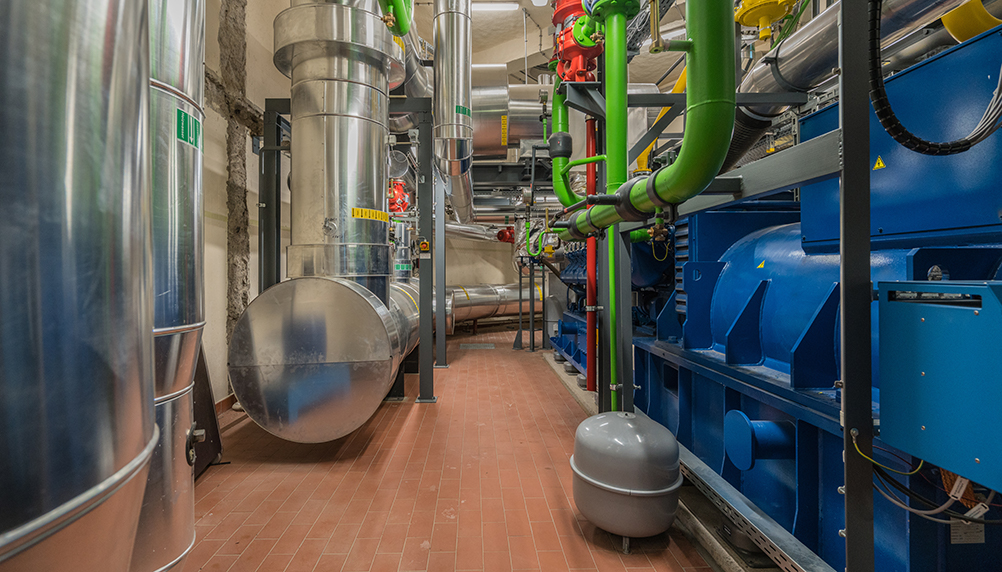# Don’t turn it off!

Tags:Following on from our look at the international and national factors effecting the current energy (price) crisis. We look at the savings of keeping your CHP running during these times.

The future has never looked so uncertain for energy suppliers; over 25 energy suppliers have ceased trading since last year (2021). Many were small firms with modest customer bases in a large market, poorly managed, underfunded or just buying energy from the wholesale market and passing it on. The biggest shock of these was the collapse of CNG, an energy provider of 27 years.

At the time of writing in February 2022, the domestic market prices for gas and electricity stood at £0.0888/kWh and £0.3282/kWh respectively; whilst the non-domestic market was at £0.0870/kWh and £0.3400/kWh. The spark spread (the difference between the gas and electricity price) is around £0.25; and may increase further, as an increase in the gas price is normally followed by an increase in the electricity price.

There’s no argument that these prices are worrying, especially those operating CHP units. However, we would advise CHP operators to keep them running, as savings can still be made.

Using the figures above, let’s look at the potential savings an operator of a 100kW CHP unit can make:

The CHP unit has the following specification:

Electrical Output 100 kW 150 kW 300 kW 17 – The CHP unit does not run during the ‘night’ period

Taking the month of January as an example, the CHP unit will operate for 527 hours and generate/consume the following:

Electricity Generated in period 52,700 kWh 79,050 kWh 158,100 kWh

Before we can start calculating the net savings of the CHP unit in the period, we must know if the CHP unit is registered under the CHPQA Programme.

If a CHP unit is registered under CHPQA Programme and meets the criteria for good quality CHP, then all the gas used and all the electricity generated is exempt from the climate change levy (CCL). We will for this example assume that the CHP unit is CHPQA registered and is classed as good quality.

For the following example, we will assume the following utility prices at site:

Electricity Rate Price £0.34000/kWh £0.08700/kWh £0.04200/kWh

The Climate Change Levy rates are currently as follows:

Gas £0.00465/kWh £0.00775/kWh

We will also assume for the following example that the gas fired back-up boiler heat efficiency is 85%.

We now have all the data we need to calculate the CHP savings. The first step is to calculate the CHP costs.

## CHP Costs

Effectively the total cost to operate the CHP, is the cost of the gas consumed and the cost of the maintenance to operate it; so we have:

Cost of CHP Gas Cost of CHP Maintenance 158,100 kWh x £0.087/kWh £13,754.70 52,700 kWh x £0.0420/kWh £2,213.40 £15,968.10

## CHP Savings

We will now look at the CHP savings. These can be separated into two (2) parts; the electricity savings and the heat savings:

### The Electricity Savings

If there was no CHP unit operating on site, then the electricity generated by the CHP unit would have to be imported from the grid and would include the cost of CCL. So the cost of importing the equivalent electricity from the grid would be:

Electricity Rate Price £0.34000/kWh £0.00775/kWh £0.34775/kWh

Therefore the total cost to import the equivalent electricity from the grid would be:

 Total Cost Equivalent Grid 52,700 kWh x £0.34775/kWh £18,326.43

### The Heat Savings

We must also take into account the heat supplied by the CHP unit; for if this was not available, this heat would have to be supplied by the site boiler(s), which would use gas to generate it.

Assuming that the boiler has a heat efficiency of 85%, then the quantity of gas used to generate the equivalent heat would be:

 Equivalent Boiler Gas 79,050 kWh/0.85 93,000 kWh

As the gas used in the boiler is not subject to a CCL exemption, then the CCL rate for gas needs to be included in the price of the gas. So the price of the boiler gas would be:

Gas Price £0.08700/kWh £0.00465/kWh £0.09165/kWh

Therefore, the total cost of the boiler gas used to replace the equivalent heat from the CHP would be:

 Total Boiler Gas Cost 93,000 kWh x £0.09165/kWh £8,523.45

So this an effective saving, as this gas does not have to be purchased to generate the heat that is being supplied by the CHP unit.

Therefore the total savings by operating the CHP unit are as follows:

Total Electricity Saving £18,326.43 £8,523.45 £26,849.88

From this we must deduct the Total CHP Operational Cost:

Total CHP Operational Cost£15,968.10

This gives us then a total nett CHP Saving of: £10,881.78

So we can see that, even with the current high gas prices and the spark spread maintained, significant savings from a CHP unit can still be made.